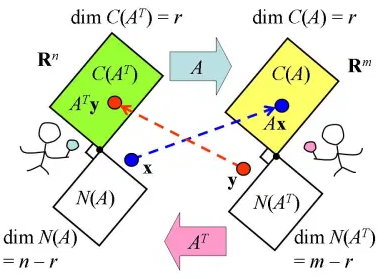# 转置矩阵#

《机器学习数学基础》第 2 章 2.3.2 节介绍了转置矩阵的基本概念，并且在之后的有关问题探讨中，经常会用到转置矩阵。为了能深入理解转置矩阵，转载参考资料  中以线性变换角度理解转置矩阵的意义的有关内容，供读者参考。## 1. 转置矩阵的定义#

$(\pmb{Ax})^{\rm{T}}\pmb{y}=\pmb{x}^{\rm{T}}\pmb{A}^{\rm{T}}\pmb{y}=\pmb{x}^{\rm{T}}(\pmb{A}^{\rm{T}}\pmb{y})$

$$m\times n$$ 的实矩阵 $$\pmb{A}$$ ，则转置矩阵 $$\pmb{A}^{\text{T}}$$ 应满足：$$(\pmb{Ax})^{\rm{T}}\pmb{y}=\pmb{x}^{\rm{T}}(\pmb{A}^{\rm{T}}\pmb{y})$$

## 参考文献#

. 线代启示录：转置矩阵的意义

. 关于内积和点积的详细内容，请参阅《机器学习数学基础》第1章1.4.2节。​